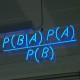## “Why are Certain Properties of Binary Relations Relatively More Common in Natural Language?”, A. Rubinstein (1996)

Everyone agrees that Rubinstein is a genius, right? In this old Econometrica of his, he applies game theory to a question of language: why do certain binary relations appear frequently – “A is to the right of B” – but others do not – “A lies clockwise from B”? Assume that languages are limited to a low number of binary relations, and that some sort of evolutionary process leads languages to tend toward the most “optimal” one.

Recall that a linear ordering is a complete, asymmetric and transitive binary relation. “To the right of” is one such linear ordering. Let the world contain a finite number of objects. A binary relation allows us to indicate – meaning to create a truth statement in predicate logic for which A is the only element in a given set making the statement true – any element of any subset of the grand set iff the relation is a binary ordering. In particular, there is a logical statement defining the “maximal element” of any set ordered in a linear ordering, and a second-maximal, and a third-maximal, etc. For this reason, we can say that linear orderings are the binary relation which is best among all possible binary relations in a language for “indicativeness”.

Rubinstein similarly proves other properties we might like a relation in a language are satisfied by linear orderings (although transitivity is not required for all of these): when a language has only one binary relation, a linear ordering is the most “precise” and a linear ordering allows a set of relations to be described with a minimal number of examples. The second property, roughly, is that if {A,B,C,D} are related by a linear ordering, and you know aRb, cRd, bRd and aRc, then you know every other possible relation among that set.

As usual, Rubinstein makes no claims that languages do in fact “naturally” find properties that are optimal: certainly English spelling is an obvious counterexample. His point is merely that language is nothing if not a coordination game, that the equilibria of a coordination games gives payoffs based on the properties of its solution, and that therefore economic and mathematic tools can be applied even to the study of language.

http://arielrubinstein.tau.ac.il/el/EL.pdf (Although this paper originally appeared in Econometrica, the ideas therein were expanded in chapter 1 of Rubinstein’s book on Economics and Language, which I have linked to.)

1.Links | Blog Pra falar de coisas says: